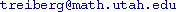Course Title: Riemannian Geometry Fall 2007 MATH 6170 - 1 Andrejs Treibergs M† 3:05-3:55 in LCB 215; W 3:05-4:55 in JWB 308. († no class on the first Mondays of the month 10-1, 11-5, 12-3.) in JWB 224.Some knowledge of differentiable manifolds would be useful (e.g. MATH 6510-6520 or consent of instructor.) Isaac Chavel, Riemannian Geometry -- A Modern Introduction, 2nd edition, Cambridge 2006

This course is useful for students of geometry, topology, geometric group theory, nonlinear analysis, general relativity and graphic design.

Abstract. Perelman's results on Hamilton's Program to solve Thurston's Geometriztion Conjecture, which implies the Poincare Conjecture, has the mathematical world extremely excited. Mathematicians expect that detailed proofs for Perelman's arguments will eventually be given.

The objective of this course is to start from basic notions from Riemannian Geometry and build to Perelman's Theorems. How does curvature influence the local and global geometry and topology of a manifold? We shall discuss basic examples such as hyperbolic space and Riemannian surfaces in considerable detail. An important tool is to use geodesic curves, which locally minimize length and whose behavior is influenced by curvature. We shall develop the intrinsic, classical and differential form notations in parallel. We shall develop some geometric analysis tools to study the Ricci Flow, a systematic deformation that splits a manifold into understandable pieces, used by Perelman.

Topics. We shall loosely follow Chavel's text for the bulk of the course. Topics include (depending on time and student's interests):

• Riemannian Metrics
• Connections. Affine & Riemannian.
• Geodesics. Minimizing properties of geodesics; convex neighborhoods; geodesic flow; exponential map. Completeness.
• Curvature; tensors.
• Calculating with moving frames.
• Sectional curvature; Ricci & scalar curvature.
• Space Forms. Cartan's Theorem on recovering the metric from curvature; hyperbolic space and its properties.
• Variation of Geodesics. First and second variation formulae for length and energy of geodesics.
• Jacobi fields.
• Theorems of Bonnet-Meyers-Synge.
• Index Theory of Geodesics. Sturm-Liouville Theory. Rauch Comparison Theorem. Hessian comparison theorem. Laplacian Comparison Theorem and applications.
• Closed Geodesics. Preissman's Theorem.
• Cut locus. Conjugate locus. Injectivity radius.
• Isometric Immersions. Second fundamental form.
• Isoperimetric inequalities.
• Toponogov Theorem. Alexandrov's Theorem. Soul Theorem.
• Sphere Theorem.
• Gromov-Hausdorff convergence.
• Collapsing.
• Ricci Flow and Hamilton's Sphere Theorem.

Last updated: 9 / 14 / 7